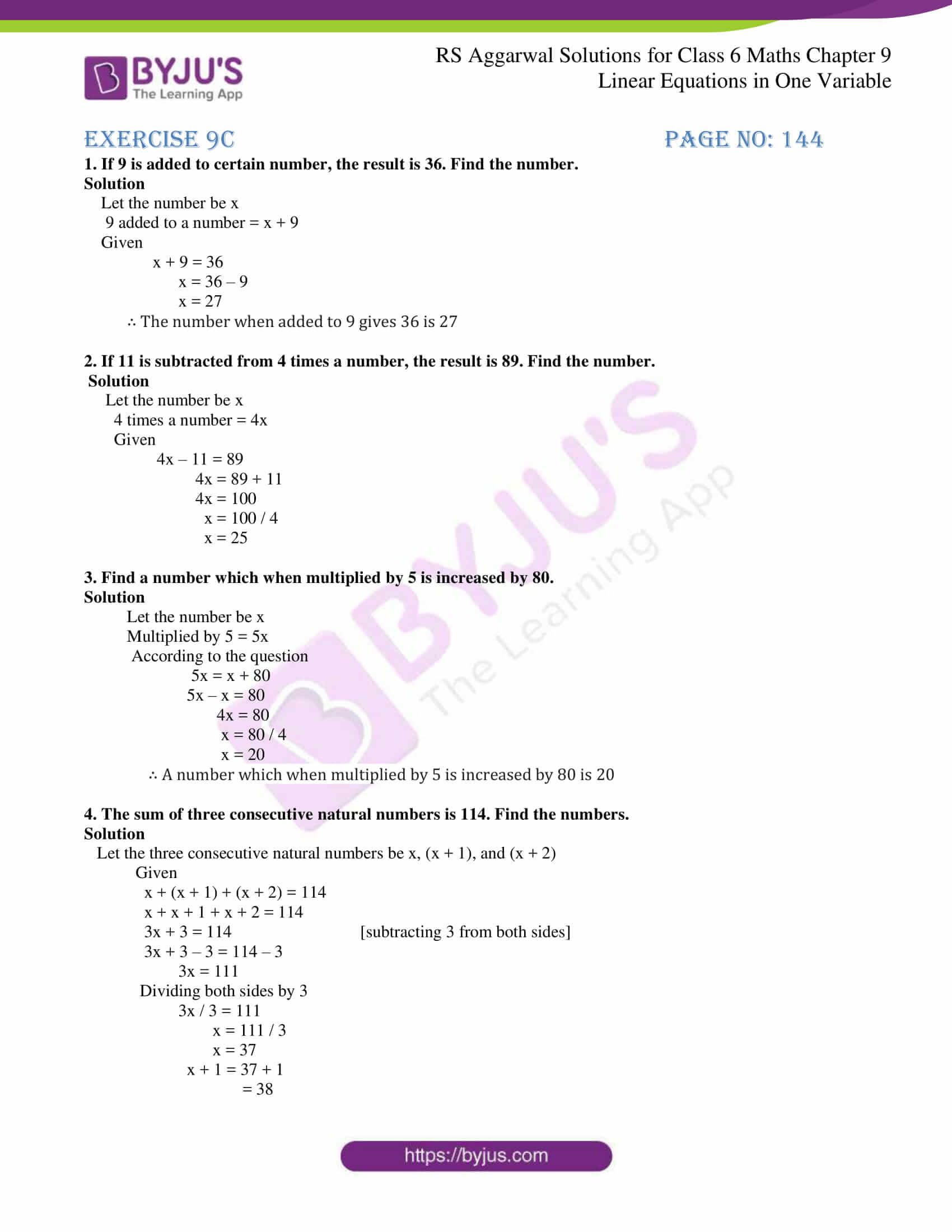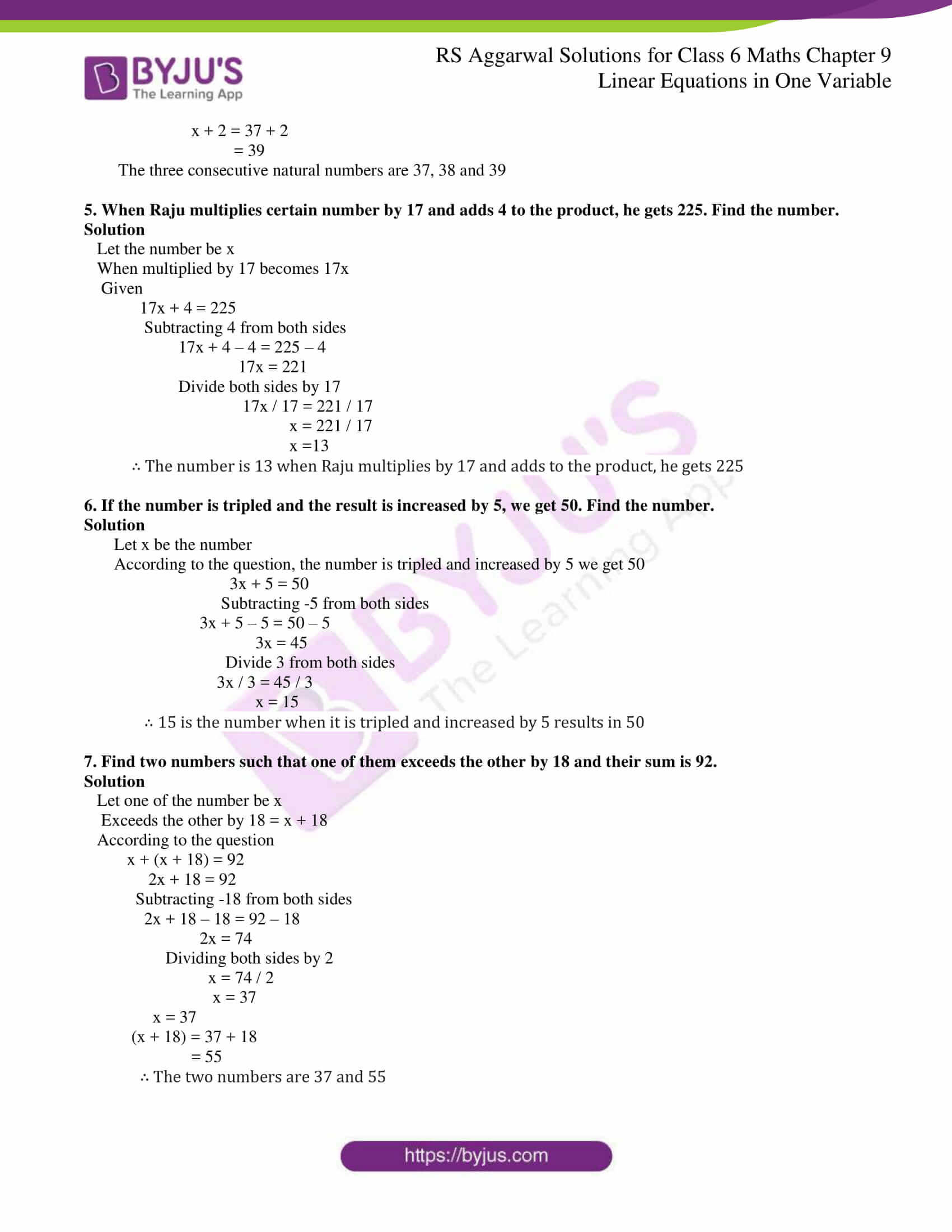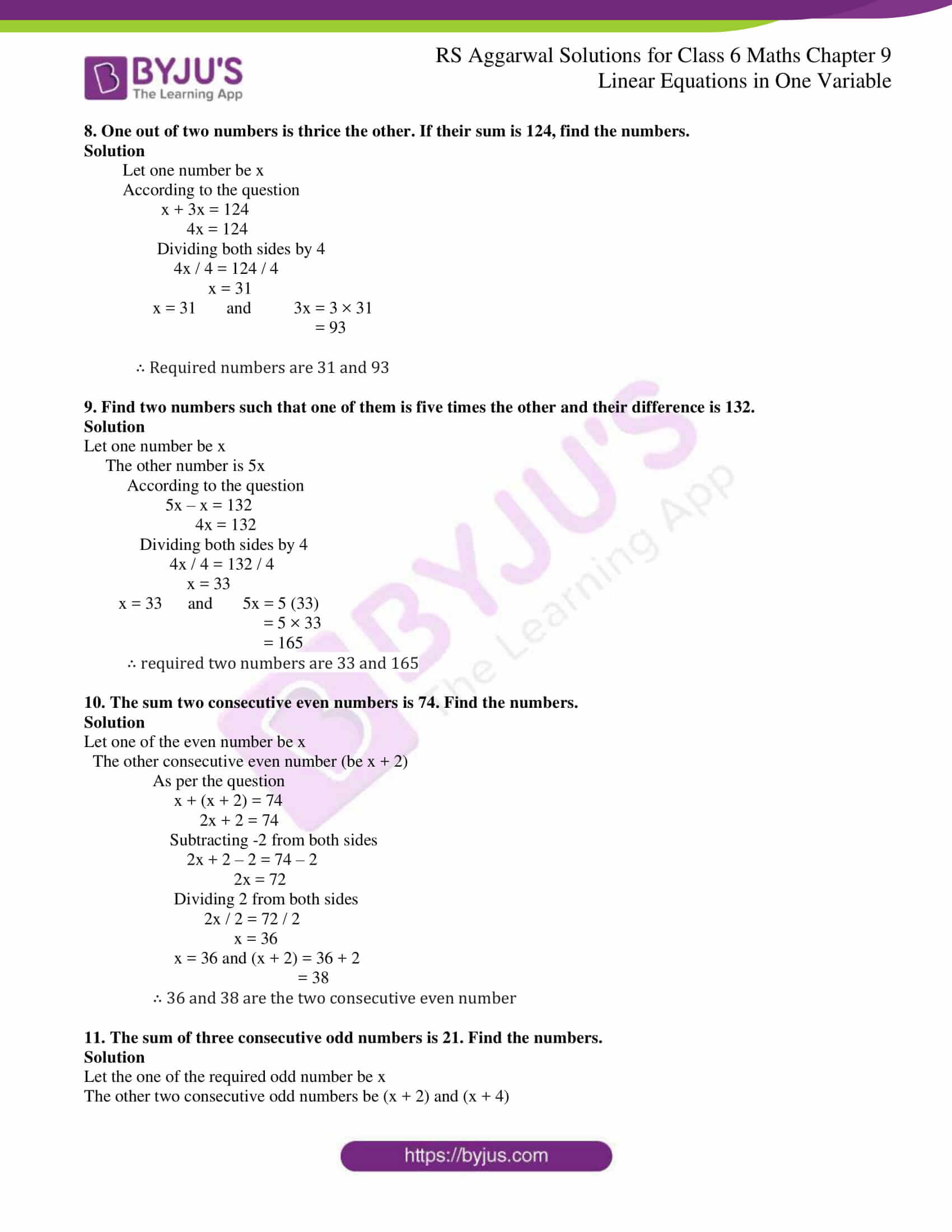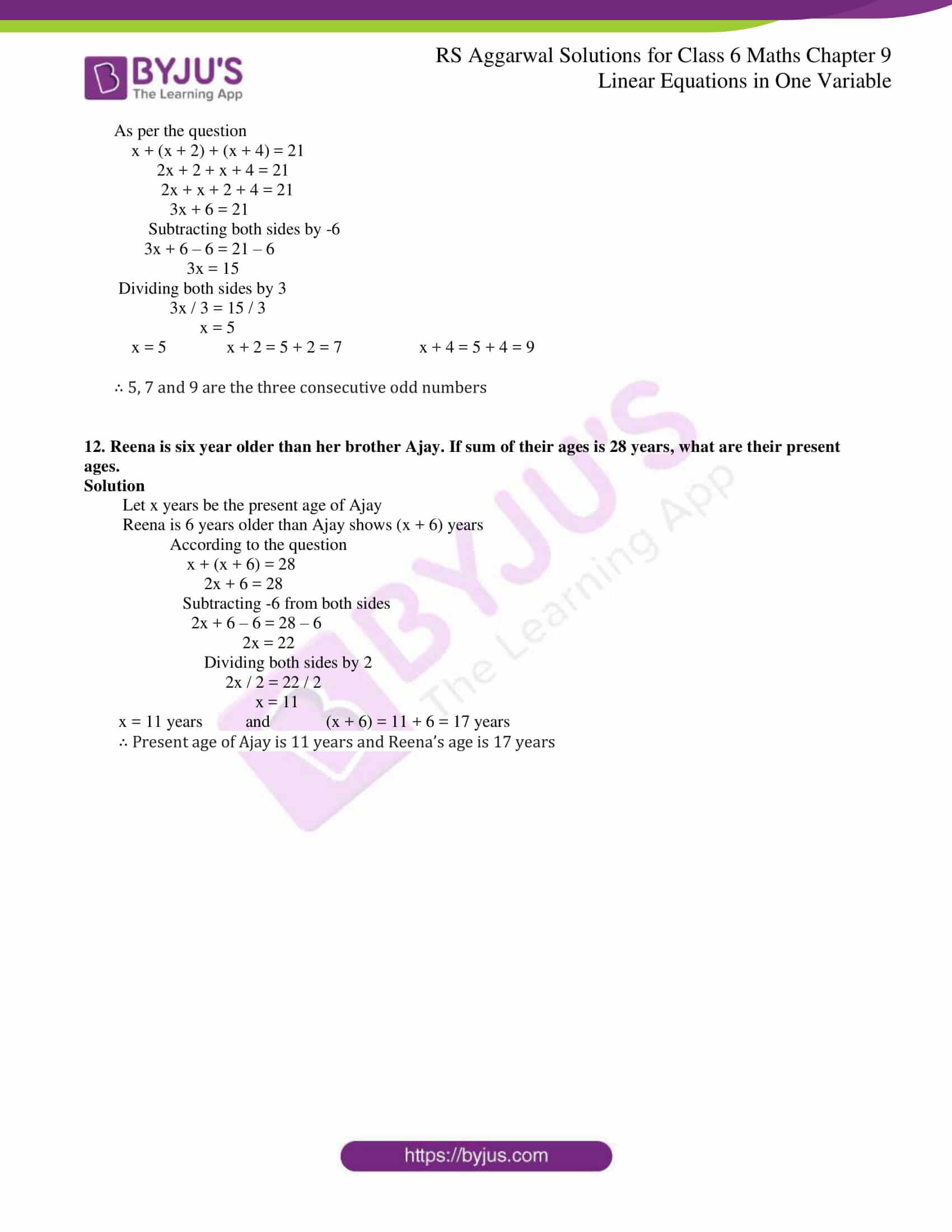# RS Aggarwal Solutions for Class 6 Chapter 9 Linear Equations in One Variable Exercise 9C

Mathematics is one of the scoring subjects which help students to obtain good overall rank in Class 6 exam. The provided PDF contains exercise-wise solutions with step by step explanations to make them easy for the students to understand. Exercise 9C explains to students about the various applications of equations in day to day life. The solutions PDF can be used as a reference guide while solving problems from RS Aggarwal textbook. To know more about the concepts which are explained here, the students can access RS Aggarwal Solutions for Class 6 Chapter 9 Linear Equations in One Variable Exercise 9C PDF.

## Download PDF of RS Aggarwal Solutions for Class 6 Chapter 9 Linear Equations in One Variable Exercise 9C### Access answers to Maths RS Aggarwal Solutions for Class 6 Chapter 9 Linear Equations in One Variable Exercise 9C

1. If 9 is added to certain number, the result is 36. Find the number.

Solution

Let the number be x

9 added to a number = x + 9

Given

x + 9 = 36

x = 36 – 9

x = 27

∴ The number when added to 9 gives 36 is 27

2. If 11 is subtracted from 4 times a number, the result is 89. Find the number.

Solution

Let the number be x

4 times a number = 4x

Given

4x – 11 = 89

4x = 89 + 11

4x = 100

x = 100 / 4

x = 25

3. Find a number which when multiplied by 5 is increased by 80.

Solution

Let the number be x

Multiplied by 5 = 5x

According to the question

5x = x + 80

5x – x = 80

4x = 80

x = 80 / 4

x = 20

∴ A number which when multiplied by 5 is increased by 80 is 20

4. The sum of three consecutive natural numbers is 114. Find the numbers.

Solution

Let the three consecutive natural numbers be x, (x + 1), and (x + 2)

Given

x + (x + 1) + (x + 2) = 114

x + x + 1 + x + 2 = 114

3x + 3 = 114 [subtracting 3 from both sides]

3x + 3 – 3 = 114 – 3

3x = 111

Dividing both sides by 3

3x / 3 = 111

x = 111 / 3

x = 37

x + 1 = 37 + 1

= 38

x + 2 = 37 + 2

= 39

The three consecutive natural numbers are 37, 38 and 39

5. When Raju multiplies certain number by 17 and adds 4 to the product, he gets 225. Find the number.

Solution

Let the number be x

When multiplied by 17 becomes 17x

Given

17x + 4 = 225

Subtracting 4 from both sides

17x + 4 – 4 = 225 – 4

17x = 221

Divide both sides by 17

17x / 17 = 221 / 17

x = 221 / 17

x =13

∴ The number is 13 when Raju multiplies by 17 and adds to the product, he gets 225

6. If the number is tripled and the result is increased by 5, we get 50. Find the number.

Solution

Let x be the number

According to the question, the number is tripled and increased by 5 we get 50

3x + 5 = 50

Subtracting -5 from both sides

3x + 5 – 5 = 50 – 5

3x = 45

Divide 3 from both sides

3x / 3 = 45 / 3

x = 15

∴ 15 is the number when it is tripled and increased by 5 results in 50

7. Find two numbers such that one of them exceeds the other by 18 and their sum is 92.

Solution

Let one of the number be x

Exceeds the other by 18 = x + 18

According to the question

x + (x + 18) = 92

2x + 18 = 92

Subtracting -18 from both sides

2x + 18 – 18 = 92 – 18

2x = 74

Dividing both sides by 2

x = 74 / 2

x = 37

x = 37

(x + 18) = 37 + 18

= 55

∴ The two numbers are 37 and 55

8. One out of two numbers is thrice the other. If their sum is 124, find the numbers.

Solution

Let one number be x

According to the question

x + 3x = 124

4x = 124

Dividing both sides by 4

4x / 4 = 124 / 4

x = 31

x = 31 and 3x = 3 × 31

= 93

∴ Required numbers are 31 and 93

9. Find two numbers such that one of them is five times the other and their difference is 132.

Solution

Let one number be x

The other number is 5x

According to the question

5x – x = 132

4x = 132

Dividing both sides by 4

4x / 4 = 132 / 4

x = 33

x = 33 and 5x = 5 (33)

= 5 × 33

= 165

∴ required two numbers are 33 and 165

10. The sum two consecutive even numbers is 74. Find the numbers.

Solution

Let one of the even number be x

The other consecutive even number (be x + 2)

As per the question

x + (x + 2) = 74

2x + 2 = 74

Subtracting -2 from both sides

2x + 2 – 2 = 74 – 2

2x = 72

Dividing 2 from both sides

2x / 2 = 72 / 2

x = 36

x = 36 and (x + 2) = 36 + 2

= 38

∴ 36 and 38 are the two consecutive even number

11. The sum of three consecutive odd numbers is 21. Find the numbers.

Solution

Let the one of the required odd number be x

The other two consecutive odd numbers be (x + 2) and (x + 4)

As per the question

x + (x + 2) + (x + 4) = 21

2x + 2 + x + 4 = 21

2x + x + 2 + 4 = 21

3x + 6 = 21

Subtracting both sides by -6

3x + 6 – 6 = 21 – 6

3x = 15

Dividing both sides by 3

3x / 3 = 15 / 3

x = 5

x = 5 x + 2 = 5 + 2 = 7 x + 4 = 5 + 4 = 9

∴ 5, 7 and 9 are the three consecutive odd numbers

12. Reena is six year older than her brother Ajay. If sum of their ages is 28 years, what are their present ages.

Solution

Let x years be the present age of Ajay

Reena is 6 years older than Ajay shows (x + 6) years

According to the question

x + (x + 6) = 28

2x + 6 = 28

Subtracting -6 from both sides

2x + 6 – 6 = 28 – 6

2x = 22

Dividing both sides by 2

2x / 2 = 22 / 2

x = 11

x = 11 years and (x + 6) = 11 + 6 = 17 years

∴ Present age of Ajay is 11 years and Reena’s age is 17 years